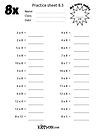# and all things relating to the eight times tables

### The Eight Times Tables

1 x 8 = 8

2 x 8 = 16

3 x 8 = 24

4 x 8 = 32

5 x 8 = 40

6 x 8 = 48

7 x 8 = 56

8 x 8 = 64

9 x 8 = 72

10 x 8 = 80

11 x 8 = 88

12 x 8 = 96

###### Note

All the answers in the eight times table are even numbers

So the last digit of the answer must always be 0, 2, 4, 6, or 8

## Division tip

If you recognise a number as belonging to a particular theme (e.g. you know that 64 is part of the Explorer Eights) then you know that 8 is a factor of 64

## free Printables###### The eight times table

Plain and straightforward, great for reference

## free practice sheets###### Sheet 8.1

Just the eight times table. Good place to start learning###### Sheet 8.2

Random order multiplication questions for the 8x table###### Sheet 8.3

4x and 8x table - double your 4x to get your 8x.

Find the links between the twoPosts are coming soon
Stay tuned...